mechanical energy to electrical energy. State the rule to be applied when you determine the direction of induced emf in a coil. State the purpose of slip rings. State the reason why no emf is induced in a rotating coil as it passes through a neutral plane. ">Custom Search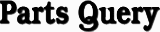Introduction to Generators and MotorsThe elementary dc generator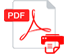DIRECT CURRENT GENERATORS

LEARNING OBJECTIVES

Upon completion of the chapter you will be able to:

• State the principle by which generators convert mechanical energy to electrical energy.
• State the rule to be applied when you determine the direction of induced emf in a coil.
• State the purpose of slip rings.
• State the reason why no emf is induced in a rotating coil as it passes through a neutral plane.
• State what component causes a generator to produce direct current rather than alternating current.
• Identify the point at which the brush contact should change from one commutator segment to the next.
• State how field strength can be varied in a dc generator.
• Describe the cause of sparking between brushes and commutator.
• State what is meant by "armature reaction."
• State the purpose of interpoles.
• Explain the effect of motor reaction in a dc generator.
• Explain the causes of armature losses.
• List the types of armatures used in dc generators.
• State the three classifications of dc generators.
• State the term that applies to voltage variation from no-load to full-load conditions and how it is expressed as a percentage.
• State the term that describes the use of two or more generators to supply a common load.
• State the purpose of a dc generator that has been modified to function as an amplidyne.

INTRODUCTION

A generator is a machine that converts mechanical energy into electrical energy by using the principle of magnetic induction. This principle is explained as follows:

Whenever a conductor is moved within a magnetic field in such a way that the conductor cuts across magnetic lines of flux, voltage is generated in the conductor.

The AMOUNT of voltage generated depends on (1) the strength of the magnetic field, (2) the angle at which the conductor cuts the magnetic field, (3) the speed at which the conductor is moved, and (4) the length of the conductor within the magnetic field.

The POLARITY of the voltage depends on the direction of the magnetic lines of flux and the direction of movement of the conductor. To determine the direction of current in a given situation, the LEFT-HAND RULE FOR GENERATORS is used. This rule is explained in the following manner.

Extend the thumb, forefinger, and middle finger of your left hand at right angles to one another, as shown in figure 1-1.

Point your thumb in the direction the conductor is being moved. Point your forefinger in the direction of magnetic flux (from north to south). Your middle finger will then point in the direction of current flow in an external circuit to which the voltage is applied.

Figure 1-1. - Left-hand rule for generators.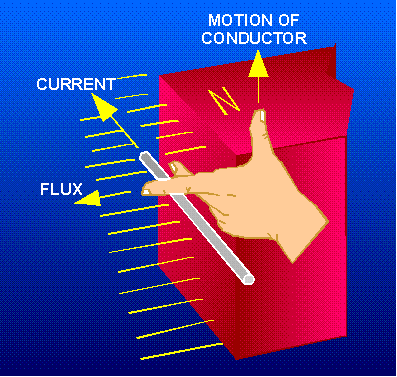THE ELEMENTARY GENERATOR

The simplest elementary generator that can be built is an ac generator. Basic generating principles are most easily explained through the use of the elementary ac generator. For this reason, the ac generator will be discussed first. The dc generator will be discussed later.

An elementary generator (fig. 1-2) consists of a wire loop placed so that it can be rotated in a stationary magnetic field. This will produce an induced emf in the loop. Sliding contacts (brushes) connect the loop to an external circuit load in order to pick up or use the induced emf.

Figure 1-2. - The elementary generator.The pole pieces (marked N and S) provide the magnetic field. The pole pieces are shaped and positioned as shown to concentrate the magnetic field as close as possible to the wire loop. The loop of wire that rotates through the field is called the ARMATURE. The ends of the armature loop are connected to rings called SLIP RINGS. They rotate with the armature. The brushes, usually made of carbon, with wires attached to them, ride against the rings. The generated voltage appears across these brushes.

The elementary generator produces a voltage in the following manner (fig. 1-3). The armature loop is rotated in a clockwise direction. The initial or starting point is shown at position A. (This will be considered the zero-degree position.) At 0 the armature loop is perpendicular to the magnetic field. The black and white conductors of the loop are moving parallel to the field. The instant the conductors are moving parallel to the magnetic field, they do not cut any lines of flux. Therefore, no emf is induced in the conductors, and the meter at position A indicates zero. This position is called the NEUTRAL PLANE. As the armature loop rotates from position A (0) to position B (90), the conductors cut through more and more lines of flux, at a continually increasing angle. At 90 they are cutting through a maximum number of lines of flux and at maximum angle. The result is that between 0 and 90, the induced emf in the conductors builds up from zero to a maximum value. Observe that from 0 to 90, the black conductor cuts DOWN through the field. At the same time the white conductor cuts UP through the field. The induced emfs in the conductors are series-adding. This means the resultant voltage across the brushes (the terminal voltage) is the sum of the two induced voltages. The meter at position B reads maximum value. As the armature loop continues rotating from 90 (position B) to 180 (position C), the conductors which were cutting through a maximum number of lines of flux at position B now cut through fewer lines. They are again moving parallel to the magnetic field at position C. They no longer cut through any lines of flux. As the armature rotates from 90 to 180, the induced voltage will decrease to zero in the same manner that it increased during the rotation from 0 to 90. The meter again reads zero. From 0 to 180 the conductors of the armature loop have been moving in the same direction through the magnetic field. Therefore, the polarity of the induced voltage has remained the same. This is shown by points A through C on the graph. As the loop rotates beyond 180 (position C), through 270 (position D), and back to the initial or starting point (position A), the direction of the cutting action of the conductors through the magnetic field reverses. Now the black conductor cuts UP through the field while the white conductor cuts DOWN through the field. As a result, the polarity of the induced voltage reverses. Following the sequence shown by graph points C, D, and back to A, the voltage will be in the direction opposite to that shown from points A, B, and C. The terminal voltage will be the same as it was from A to C except that the polarity is reversed (as shown by the meter deflection at position D). The voltage output waveform for the complete revolution of the loop is shown on the graph in figure 1-3.

Figure 1-3. - Output voltage of an elementary generator during one revolution.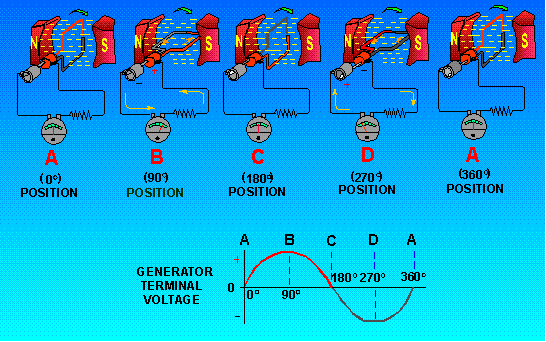Q.1 Generators convert mechanical motion to electrical energy using what principle?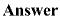Q.2 What rule should you use to determine the direction of induced emf in a coil?Q.3 What is the purpose of the slip rings?Q.4 Why is no emf induced in a rotating coil when it passes through the neutral plane?Integrated Publishing, Inc. - A (SDVOSB) Service Disabled Veteran Owned Small Business